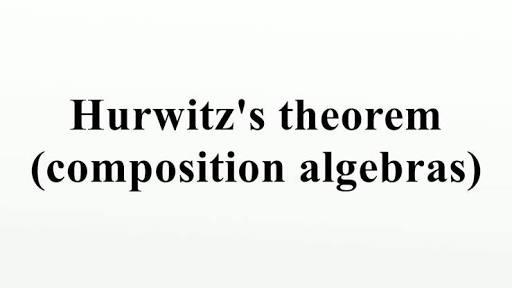# What Do You Know About Hurwitz's Theorem On Composition Algebras?

10 Questions | Total Attempts: 105SettingsHurwitz's theorem on composition algebra was developed by mathematician Adolf Hurwitz but was later published officially after his death. The theorem has been used to solve numerous mathematical problems and has been established as one of the most important theorems. To learn more about this theorem, practice the quiz highlighted below.

• 1.
When was the theorem published posthumously?
• A.

1922

• B.

1923

• C.

1924

• D.

1925

• 2.
Which form defines the hormomorphism?
• A.

• B.

Algebraic form

• C.

Exponential form

• D.

Geometric form

• 3.
The hormomorphism is defined into what?
• A.

Positive real number

• B.

Negative real number

• C.

Integer

• D.

Complex number

• 4.
Which part of the algebra is involved in the theorem?
• A.

Zero part

• B.

Irrational numbers

• C.

Non-zero part

• D.

Rational numbers

• 5.
According to the theorem, the algebra is NOT isomorphic to which of the following?
• A.

Real numbers

• B.

Complex numbers

• C.

Integers

• D.

Octonions

• 6.
The Hurwitz algebras are an example of what?
• A.

Composition algebras

• B.

Non-composition algebras

• C.

Real algebras

• D.

Irrational algebras

• 7.
Which of these is the correct representation of the theorem?
• A.

Q ( a b) = q ( a)  q ( b)

• B.

Q ( a b) = q ( a)  / q ( b)

• C.

Q ( a b) = q ( a) ( b)

• D.

Q ( a b) = ( a) ( b)

• 8.
The multiplicative formulas used for the sum of squares can occur in each of the following dimensions except which one?
• A.

1

• B.

2

• C.

4

• D.

5

• 9.
Which of these has the theorem never been applied to?
• A.

Classical group

• B.

Crystallized group

• C.

Algebraic topography

• D.

Homotopy group

• 10.
In the theorem, what does q represent?
• A.

Irrational numbers

• B.

Real positive numbers

• C.

Negative-definate

• D.

Positive-definate

Related TopicsBack to top